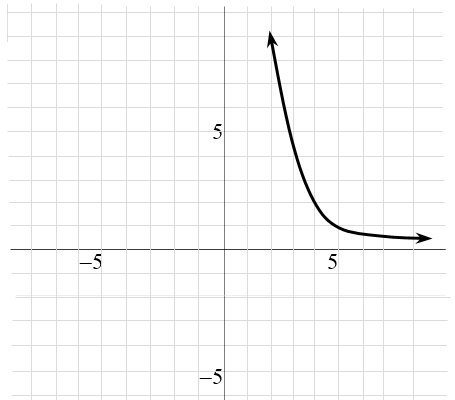### Home > INT2 > Chapter 2 > Lesson 2.3.2 > Problem2-97

2-97.

Write the equation of an exponential function that passes through the points $(0, 32)$ and $(3, 4)$.

Here is a visual of the equation's graph.

You know $32$ is the coefficient from the point $(0, 32)$.
What number raised to the $3$rd power and multiplied by $32$,
equals $4$?

$f(x) \ = \ 32\left( \frac{1}{2} \right)^x$